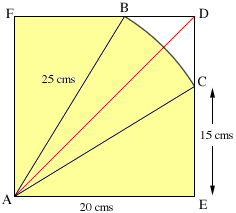My name: Jacky Level of question. Grade 10-11 Secondary school Who is asking the question: Student I just saw a problem one day in the textbook in which our teacher says it is a problem slightly harder than grade 10 level. Here is the problem: (It is more helpful to draw a diagram) A square with a dimension 20 by 20cm. and a quarter of the circle with the radius of 25cm (A quater of a circle is created by 2 cuts that are perpendicular bisectors of each other where the intersecting point is at the centre of the circle). With these 2 pieces, the 2 pieces are placed over each other in which the 90o angle of the quarter circle matches with one of the right angles on the square. Now, calculate the overlapping area of the 2 figures. Thanks for taking the time to think about this question. Hi Jacky, I think that the problem is a little harder than the grade 10 level as I think you need to use some trigonometry to solve it. The overlapping region (the part shaded yellow in the diagram) can be seen as two triangles (ABF and AEC) and a sector of a circle (ACB).Each of the triangles has area 1/2 x base x height = 1/2 x 20 x 15 = 150 square cms. and hence the area of the two triangles is 300 square cms. The area of a sector of a circle is 1/2 r2 theta where theta is the angle at the center of the circle measured in radians. Divide the sector in half by the line from A to D and then the area of the sector is 2[1/2 252 angle DAC] Fianlly angle DAC = angle DAE - angle CAE. Angle DAE is 45o or in radians pi/4. The easiest way to describe angle CAE is to use trigonometry and say that it is the angle whose tangent is 15/20 = 3/4. This angle is sometime written arctan(3/4). Thus the area of the overlapping region is 300 + 625(pi/4 - arctan(3/4)) which is approximately 388.69 square centimeters. Cheers, Chris and Harley Go to Math Central